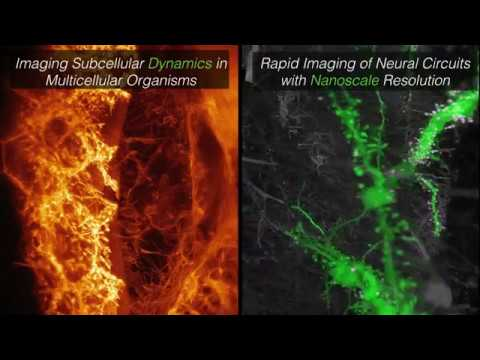# dask-image: A library for distributed image processing¶

John Kirkham (@jakirkham)

# Typical image processing use cases¶https://github.com/ageitgey/face_recognition

# Typical image processing use cases¶

• Commodity cameras
• Color images
• Fit in-memory
• Generic images of recognizable scenes
• Various successful algorithms

# Large image processing use cases¶# Large image processing use cases¶

• Specialized instruments
• Monochrome to multispectral
• Does not fit in-memory
• Domain specialists understand data
• Complex pipelines needed for analysis

# Working with large image data is hard¶

• Data size limits scientists
• Domain knowledge limits technologists

# Common workflows¶

• Batch Processing
• Large field of view

# Common workflows - Batch Processing¶

for each_fn in myfiles:
a_chunk = load(each_fn)
a_cleaned = cleanup(a_chunk)
a_mask = threshold(a_cleaned)
a_labeled = label(a_mask)
save(a_labeled)


# Common workflows - Large image¶

# Repeated for each op
for each_slice in regions:
larger_slice, cropped_slice = add_overlap(each_slice, cleanup_overlap)
a_larger = load(larger_slice)
a_large_cleaned = cleanup(a_larger)
a = a_large_cleaned[cropped_slice]
save(a)


# What are the challenges with these?¶

for each_fn in myfiles:            # <--- Not parallel
a_chunk = load(each_fn)
a_cleaned = cleanup(a_chunk)
a_mask = threshold(a_cleaned)
a_labeled = label(a_mask)
save(a_labeled)

for each_fn in myfiles:            # <--- Not parallel
a_chunk = load(each_fn)
a_cleaned = cleanup(a_chunk)   # <--- Not inspectable
a_mask = threshold(a_cleaned)
a_labeled = label(a_mask)
save(a_labeled)

for each_fn in myfiles:            # <--- Not parallel
a_chunk = load(each_fn)
a_cleaned = cleanup(a_chunk)   # <--- Not inspectable
a_mask = threshold(a_cleaned)  # <--- Not swappable
a_labeled = label(a_mask)
save(a_labeled)

for each_fn in myfiles:            # <--- Not parallel
a_chunk = load(each_fn)
a_cleaned = cleanup(a_chunk)   # <--- Not inspectable
a_mask = threshold(a_cleaned)  # <--- Not swappable
a_labeled = label(a_mask)
save(a_labeled)                # <--- Not interactive

# Repeated for each op             # <--- Higher overhead for complex ops
for each_slc in regions:
larger_slice, cropped_slice = get_cleanup_overlap(each_slice)
a_larger = load(larger_slice)
a_large_cleaned = cleanup(a_larger)
a = a_large_cleaned[cropped_slice]
save(a)


# This workflow presents challenges¶

• Fixing each step increases complexity
• Challenging to maintain
• Hard to learn
• Not very reusable

## But operate at scale¶1. Dask Array with map_blocks and map_overlap
2. Dask Image (new!)

# Loading image data¶

import dask.array as da
from dask_image.imread import imread

a = da.block([
[imread("images/fn00.tiff"), imread("images/fn01.tiff")],
[imread("images/fn10.tiff"), imread("images/fn11.tiff")],
])


Read more here: https://blog.dask.org/2019/06/20/load-image-data

# Batch Processing (Revisited)¶

a_cleaned = a.map_blocks(cleanup)
a_mask = a_cleaned.map_blocks(threshold)
a_labeled = a_mask.map_blocks(label)

for each_fn in myfiles:
a_chunk = load(each_fn)
a_cleaned = cleanup(a_chunk)
a_mask = threshold(a_cleaned)
a_labeled = label(a_mask)
save(a_labeled)


# Large Image (Revisited)¶

a_cleaned = a.map_overlap(cleanup, cleanup_overlap)
a_mask = a_cleaned.map_overlap(threshold, threshold_overlap)
a_labeled = a_mask.map_overlap(label, label_overlap)

# Repeated for each op
for each_slice in regions:
larger_slice, cropped_slice = add_overlap(each_slice, cleanup_overlap)
a_larger = load(larger_slice)
a_large_cleaned = cleanup(a_larger)
a = a_large_cleaned[cropped_slice]
save(a)


# How can we improve this workflow?¶

• .map_blocks for batch
• .map_overlap for large images

# Are we done?¶

• What about reusability?
• How do we engage domain specialists?
• By making a library using common API :)

# Smoothing Use Case¶# Smoothing Use Case¶

from scipy.ndimage import uniform_filter

uniform_filter(a, 10)


# Smoothing Use Case¶

def uniform_filter(input,
size=3,
mode='reflect',
cval=0.0,
origin=0):
size = _utils._get_size(input.ndim, size)
depth = _utils._get_depth(size, origin)

depth, boundary = _utils._get_depth_boundary(input.ndim, depth, "none")

result = input.map_overlap(
scipy.ndimage.filters.uniform_filter,
depth=depth,
boundary=boundary,
dtype=input.dtype,
size=size,
mode=mode,
cval=cval,
origin=origin
)

return result


# scipy.ndimage coverage¶

Function name SciPy ndimage dask-image
affine_transform X
binary_closing X X
binary_dilation X X
binary_erosion X X
binary_fill_holes X
binary_hit_or_miss X
binary_opening X X
binary_propagation X
black_tophat X
center_of_mass X X
convolve X X
convolve1d X
correlate X X
correlate1d X
distance_transform_bf X
distance_transform_cdt X
distance_transform_edt X
extrema X X
find_objects X
fourier_ellipsoid X
fourier_gaussian X X
fourier_shift X X
fourier_uniform X X
gaussian_filter X X
gaussian_filter1d X
gaussian_gradient_magnitude X X
gaussian_laplace X X
generate_binary_structure X
generic_filter X X
generic_filter1d X
generic_gradient_magnitude X
generic_laplace X
geometric_transform X
grey_closing X
grey_dilation X
grey_erosion X
grey_opening X
histogram X X
imread X X
iterate_structure X
label X X
labeled_comprehension X X
laplace X X
map_coordinates X
maximum X X
maximum_filter X X
maximum_filter1d X
maximum_position X X
mean X X
median X X
median_filter X X
minimum X X
minimum_filter X X
minimum_filter1d X
minimum_position X X
morphological_gradient X
morphological_laplace X
percentile_filter X X
prewitt X X
rank_filter X X
rotate X
shift X
sobel X X
spline_filter X
spline_filter1d X
standard_deviation X X
sum X X
uniform_filter X X
uniform_filter1d X
variance X X
watershed_ift X
white_tophat X
zoom X

# Future Work¶

• Adding needed functions to the API
• Working closely with the community
• Handling generalized arrays
• Exploring GPUs for similar operations

# Getting started¶

### Conda¶

conda install -c conda-forge dask-image

### Pip¶

pip install dask-image